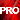##Create the 'perfect' pizza through this mathematical formulaWhat do you do if you're a mathematician with a love of pizza? You create an equation that describes the perfect pizza, of course! Eugenia Cheng of the University of Sheffield in the UK did such a thing, coming up with the formula below.Reply With Quote